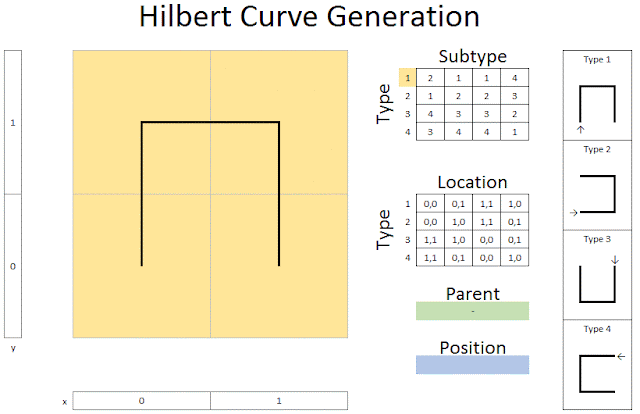## Wednesday, February 22, 2017

### I hope you find this blog enjoyable and helpful. Support it with a small donation.

I've been researching something that requires a Hilbert curve and I thought I'd share how to generate the path and also move between the index and coordinates of points.

For those of you unfamiliar with Hilbert curves let me quickly explain what one is and why you would want to use one.  The curve covers every point in a square with side length 2^n by moving up, down, left and right, starting at one corner and ending at an adjacent corner.  You could accomplish the same requirements by just scanning back and forth across the rows, but the advantage the Hilbert curve has is that nearby points on the 2D grid are generally also near each other on the curve.  If you were to scan back and forth you get a lot of points that are directly beside each other in 2D space but very far apart on the curve.

So why would you want to use one?  They're great for turning 2D areas into 1D streams of data while maintaining locality.  This comes in handy in image processing.  Depending on what you want to do, this may make the processing a lot easier.

Generation of the curve can be done recursively by first selecting an initial shape type and then using tables of sub-types and locations to generate the next stage.  In the animation below the initial shape is type 1.  When you go to the sub-type table you see that type 1 is replaced with types 2, 1, 1, and 4 in that order.  The position of each sub-type is defined by the parent type.  As the parent is type 1 the locations of the sub-types from the location table are:

2 at (0, 0)
1 at (0, 1)
1 at (1, 1)
4 at (1, 0)

The location table can then be used to find the coordinates of the sub-types.  As binary coordinates are used, the x and y coordinates of the sub-type can be appended to its coordinates to find the new sub-coordinates.   For example, the coordinates of the type 4 sub-type at position (1, 0) above are (1, 1) (0, 1) (0, 0) (1, 0).  Appending these to the coordinates of (1, 0) you find the new sub coordinates (11, 01) (10, 01) (10, 00) (11, 00).  Trust me it's confusing at first but after a while it all makes complete sense.  I'm still trying to come up with appropriate terminology for the process.  These tables are from a paper

It's a little confusing at first but I recommend reading that to help understand the process.Recursive Hilbert Curve Generation

What if you don't want to generate the full curve and just want to know where along the curve a point lays or where a point on the curve is in 2D space?  Let's use the below example to demonstrate this.44th Point at location (111, 101)

The above image shows the 44th point of the third stage at (111, 101).  It's actually the 45th point but we start counting at  0.  We add an index row to the tables provided in the above paper to make the process easier.Generation Tables

We start by converting the index to a binary number with 2 bits for every stage.  In this case it's the third stage, so we get 6 bits.  These bits are placed two at a time on rows under the index column.  We also define the initial shape as type 1.  From this, the sub-type and location for the first row can be found.  The sub-type becomes the type for the next row.  This process is repeated two more times until we have three locations.  To get the final location coordinates, just append the x and y coordinates downwards to get (111, 101).Converting an Index to a location

To go from coordinate to index, split the location up by removing the highest bit from the x and y coordinate for each line.  As the start type is known, the index can be found.  This index is then used to find the sub-type for the next row.  This is repeated two more times.  The indices are then concatenated to get 101100 in binary which is 44.Converting a location to an index

I hope this helped to explain the process.  Trust me, even if it's still confusing, having diagrams and a couple of hopefully easy to follow examples will help.